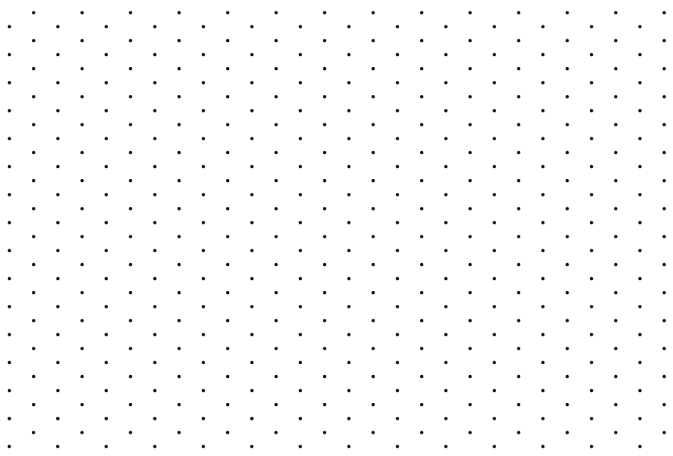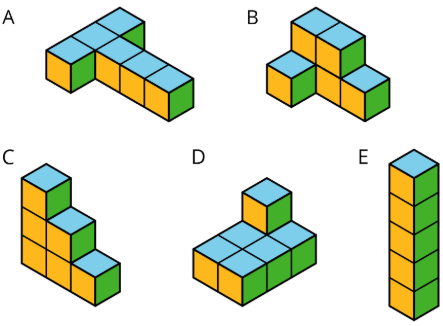$$\newcommand{\id}{\mathrm{id}}$$ $$\newcommand{\Span}{\mathrm{span}}$$ $$\newcommand{\kernel}{\mathrm{null}\,}$$ $$\newcommand{\range}{\mathrm{range}\,}$$ $$\newcommand{\RealPart}{\mathrm{Re}}$$ $$\newcommand{\ImaginaryPart}{\mathrm{Im}}$$ $$\newcommand{\Argument}{\mathrm{Arg}}$$ $$\newcommand{\norm}{\| #1 \|}$$ $$\newcommand{\inner}{\langle #1, #2 \rangle}$$ $$\newcommand{\Span}{\mathrm{span}}$$

# 1.5.5: Distinguishing Between Surface Area and Volume

•• Contributed by Illustrative Mathematics
• OpenUp Resources
$$\newcommand{\vecs}{\overset { \rightharpoonup} {\mathbf{#1}} }$$ $$\newcommand{\vecd}{\overset{-\!-\!\rightharpoonup}{\vphantom{a}\smash {#1}}}$$$$\newcommand{\id}{\mathrm{id}}$$ $$\newcommand{\Span}{\mathrm{span}}$$ $$\newcommand{\kernel}{\mathrm{null}\,}$$ $$\newcommand{\range}{\mathrm{range}\,}$$ $$\newcommand{\RealPart}{\mathrm{Re}}$$ $$\newcommand{\ImaginaryPart}{\mathrm{Im}}$$ $$\newcommand{\Argument}{\mathrm{Arg}}$$ $$\newcommand{\norm}{\| #1 \|}$$ $$\newcommand{\inner}{\langle #1, #2 \rangle}$$ $$\newcommand{\Span}{\mathrm{span}}$$ $$\newcommand{\id}{\mathrm{id}}$$ $$\newcommand{\Span}{\mathrm{span}}$$ $$\newcommand{\kernel}{\mathrm{null}\,}$$ $$\newcommand{\range}{\mathrm{range}\,}$$ $$\newcommand{\RealPart}{\mathrm{Re}}$$ $$\newcommand{\ImaginaryPart}{\mathrm{Im}}$$ $$\newcommand{\Argument}{\mathrm{Arg}}$$ $$\newcommand{\norm}{\| #1 \|}$$ $$\newcommand{\inner}{\langle #1, #2 \rangle}$$ $$\newcommand{\Span}{\mathrm{span}}$$

## Lesson

Let's contrast surface area and volume.

Exercise $$\PageIndex{1}$$: Attributes and Their Measures

For each quantity, choose one or more appropriate units of measurement.

For the last two, think of a quantity that could be appropriately measured with the given units.

Quantities

1. Perimeter of a parking lot:
2. Volume of a semi truck:
3. Surface area of a refrigerator:
4. Length of an eyelash:
5. Area of a state:
6. Volume of an ocean:
7. ________________________: miles
8. ________________________: cubic meters

Units

• millimeters (mm)
• feet (ft)
• meters (m)
• square inches (sq in)
• square feet (sq ft)
• square miles (sq mi)
• cubic kilometers (cu km)
• cubic yards (cu yd)

Exercise $$\PageIndex{2}$$: Building with 8 Cubes

This applet has 16 cubes in its hidden stack. Build two different shapes using 8 cubes for each.

For each shape, determine the following information and write it on a sticky note.

• Give a name or a label (e.g., Mae’s First Shape or Eric’s Steps).
• Determine its volume.
• Determine its surface area.

Exercise $$\PageIndex{3}$$: Comparing Prisms Without Building Them

Three rectangular prisms each have a height of 1 cm.

• Prism A has a base that is 1 cm by 11 cm.
• Prism B has a base that is 2 cm by 7 cm.
• Prism C has a base that is 3 cm by 5 cm.
1. Find the surface area and volume of each prism. Use the dot paper to draw the prisms, if needed.Figure $$\PageIndex{1}$$
1. Analyze the volumes and surface areas of the prisms. What do you notice? Write 1 or 2 observations about them.

Can you find more examples of prisms that have the same surface areas but different volumes? How many can you find?

### Summary

Length is a one-dimensional attribute of a geometric figure. We measure lengths using units like millimeters, centimeters, meters, kilometers, inches, feet, yards, and miles.Figure $$\PageIndex{2}$$

Area is a two-dimensional attribute. We measure area in square units. For example, a square that is 1 centimeter on each side has an area of 1 square centimeter.Figure $$\PageIndex{3}$$

Volume is a three-dimensional attribute. We measure volume in cubic units. For example, a cube that is 1 kilometer on each side has a volume of 1 cubic kilometer.Figure $$\PageIndex{4}$$

Surface area and volume are different attributes of three-dimensional figures. Surface area is a two-dimensional measure, while volume is a three-dimensional measure.

Two figures can have the same volume but different surface areas. For example:

• A rectangular prism with side lengths of 1 cm, 2 cm, and 2 cm has a volume of 4 cu cm and a surface area of 16 sq cm.
• A rectangular prism with side lengths of 1 cm, 1 cm, and 4 cm has the same volume but a surface area of 18 sq cm.Figure $$\PageIndex{5}$$: On left, rectangular prism with side lengths of 1 centimeter, 2 centimeters, and 2 centimeters has a volume of 4 cubic centimeters and a surface area of 16 square centimeters. On right, rectangular prism with side lengths of 1 centimeter, 1 centimeter, and 4 centimeters has the same volume but a surface area of 18 square centimeters.

Similarly, two figures can have the same surface area but different volumes.

• A rectangular prism with side lengths of 1 cm, 1 cm, and 5 cm has a surface area of 22 sq cm and a volume of 5 cu cm.
• A rectangular prism with side lengths of 1 cm, 2 cm, and 3 cm has the same surface area but a volume of 6 cu cm.Figure $$\PageIndex{6}$$: On left, rectangular prism with side lengths of 1 centimeter, 1 centimeter, and 5 centimeters has a surface area of 22 square centimeters and a volume of 5 cubic centimeters. On right, rectangular prism with side lengths of 1 centimeter, 2 centimeters, and 3 centimeters has the same surface area but a volume of 6 cubic centimeters.

### Glossary Entries

Definition: Base (of a Prism or Pyramid)

The word base can also refer to a face of a polyhedron.

A prism has two identical bases that are parallel. A pyramid has one base.

A prism or pyramid is named for the shape of its base.Figure $$\PageIndex{7}$$: The figure on the left is labeled pentagonal prism. There are two identical pentagons on the top and bottom. Each vertex of a pentagon is connected by a vertical segment to the corresponding vertex of the other pentagons. The pentagons are each shaded, with the word base pointing to each. The figure on the right is labeled hexagonal pyramid. There is a hexagon on the bottom shaded green. From a point above the hexagon extend 6 segments, each connected to a vertex of the hexagon.

Definition: Face

Each flat side of a polyhedron is called a face. For example, a cube has 6 faces, and they are all squares.

Definition: Net

A net is a two-dimensional figure that can be folded to make a polyhedron.

Here is a net for a cube.Figure $$\PageIndex{9}$$

Definition: Polyhedron

A polyhedron is a closed, three-dimensional shape with flat sides. When we have more than one polyhedron, we call them polyhedra.

Here are some drawings of polyhedra.Figure $$\PageIndex{10}$$

Definition: Prism

A prism is a type of polyhedron that has two bases that are identical copies of each other. The bases are connected by rectangles or parallelograms.

Here are some drawings of prisms.Figure $$\PageIndex{11}$$

Definition: Pyramid

A pyramid is a type of polyhedron that has one base. All the other faces are triangles, and they all meet at a single vertex.

Here are some drawings of pyramids.Figure $$\PageIndex{12}$$

Definition: Surface Area

The surface area of a polyhedron is the number of square units that covers all the faces of the polyhedron, without any gaps or overlaps.

For example, if the faces of a cube each have an area of 9 cm2, then the surface area of the cube is $$6\cdot 9$$, or 54 cm2.

Definition: Volume

Volume is the number of cubic units that fill a three-dimensional region, without any gaps or overlaps.

For example, the volume of this rectangular prism is 60 units3, because it is composed of 3 layers that are each 20 units3.Figure $$\PageIndex{13}$$

## Practice

Exercise $$\PageIndex{4}$$

Match each quantity with an appropriate unit of measurement.

1. The surface area of a tissue box
2. The amount of soil in a planter box
3. The area of a parking lot
4. The length of a soccer field
5. The volume of a fish tank
1. Square meters
2. Yards
3. Cubic inches
4. Cubic feet
5. Square centimeters

Exercise $$\PageIndex{5}$$

Here is a figure built from snap cubes.Figure $$\PageIndex{14}$$
1. Find the volume of the figure in cubic units.
2. Find the surface area of the figure in square units.
3. True or false: If we double the number of cubes being stacked, both the volume and surface area will double. Explain or show how you know.

Exercise $$\PageIndex{6}$$

Lin said, “Two figures with the same volume also have the same surface area.”

1. Which two figures suggest that her statement is true?
2. Which two figures could show that her statement is not true?Figure $$\PageIndex{15}$$: 5 figures composed of unit cubes, labeled A, B, C, D, E. A is a T shape, 3 cubes across, 3 cubes down. B, 2 cubes stacked on top of 3 cubes, 1 additional cube in front. C, 1 cube stacked on top of 2 cubes stacked on top of 3 cubes. D. 2 by 4 cubes laying flat with 1 additional cube stacked on top. E. 6 cubes stacked on top of one another, 1 by 1 by 6.

Exercise $$\PageIndex{7}$$

Draw a pentagon (five-sided polygon) that has an area of 32 square units. Label all relevant sides or segments with their measurements, and show that the area is 32 square units.

(From Unit 1.4.1)

Exercise $$\PageIndex{8}$$

1. Draw a net for this rectangular prism.Figure $$\PageIndex{16}$$
1. Find the surface area of the rectangular prism.

(From Unit 1.5.4)